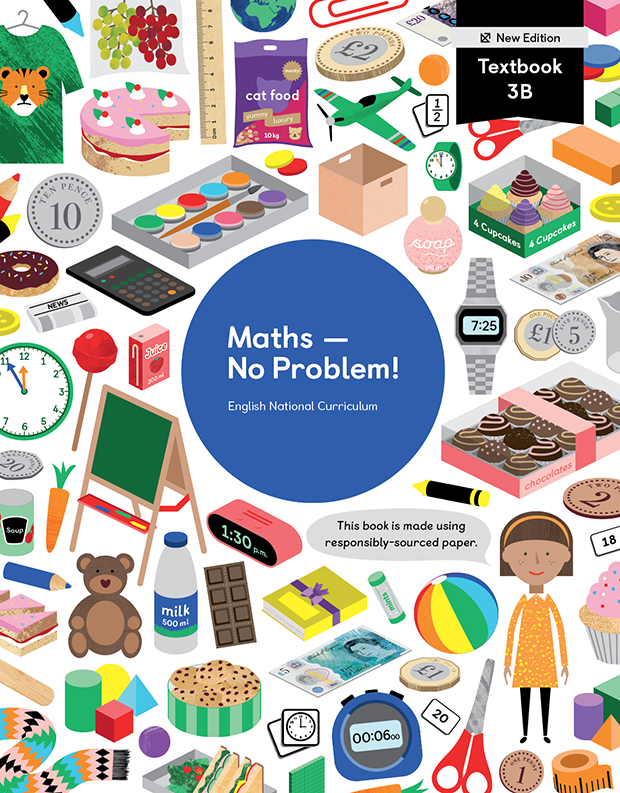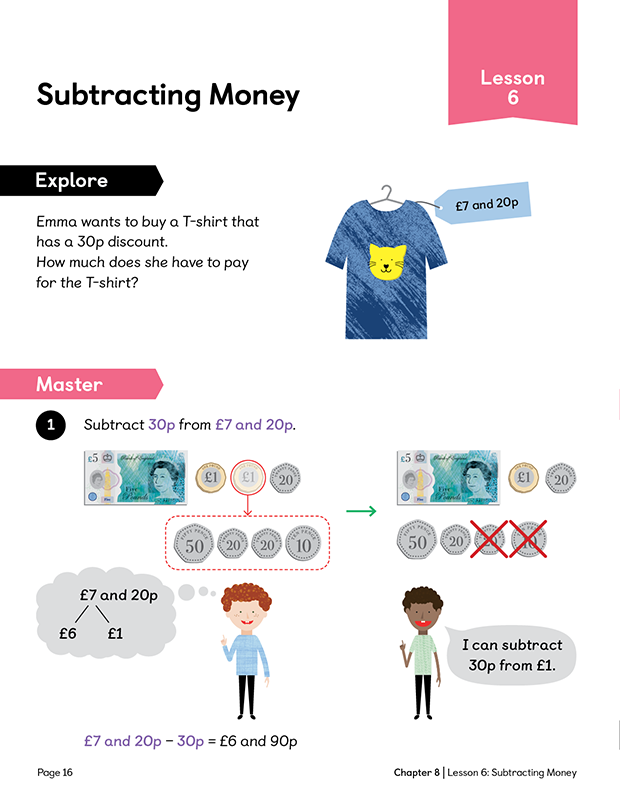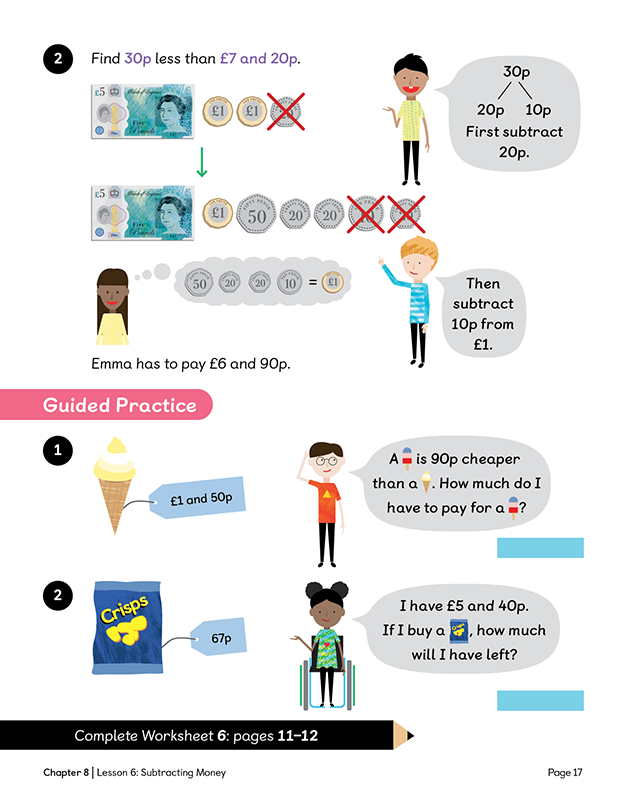Textbook 3B New Edition# Maths — No Problem!Textbook 3B New Edition

## £11.49

ISBN: 9781913458560
Pages: 220
Availability:
In stock
Qty:

• Lesson 1 - Counting MoneyTo be able to find a total amount of money by counting on.
• Lesson 2 - Showing Amounts of MoneyTo be able to show amounts of money using different combinations of coins.
• Lesson 3 - Adding MoneyTo be able to add pounds and pence without renaming.
• Lesson 4 - Adding MoneyTo be able to add pounds and pence with renaming.
• Lesson 5 - Subtracting MoneyTo be able to subtract pounds and pence without renaming.
• Lesson 6 - Subtracting MoneyTo be able to subtract pounds and pence with renaming.
• Lesson 7 - Subtracting MoneyTo be able to compare amounts of money and find the difference by subtracting.
• Lesson 8 - Calculating ChangeTo be able to find the amount of change left from a given amount of money after a purchase.
• Lesson 9 - Solving Word ProblemsTo be able to solve word problems involving addition and subtraction of money.
• Lesson 10 - Solving Word ProblemsTo be able to solve multi-step word problems involving addition and subtraction of money.
• Chapter ConsolidationTo reflect on learning from Chapter 8.

## Author(s)

Consultant Editors: Dr Wong Khoon Yoong, Dr Julie Alderton
Series Editors: Adam Gifford, Andy Psarianos